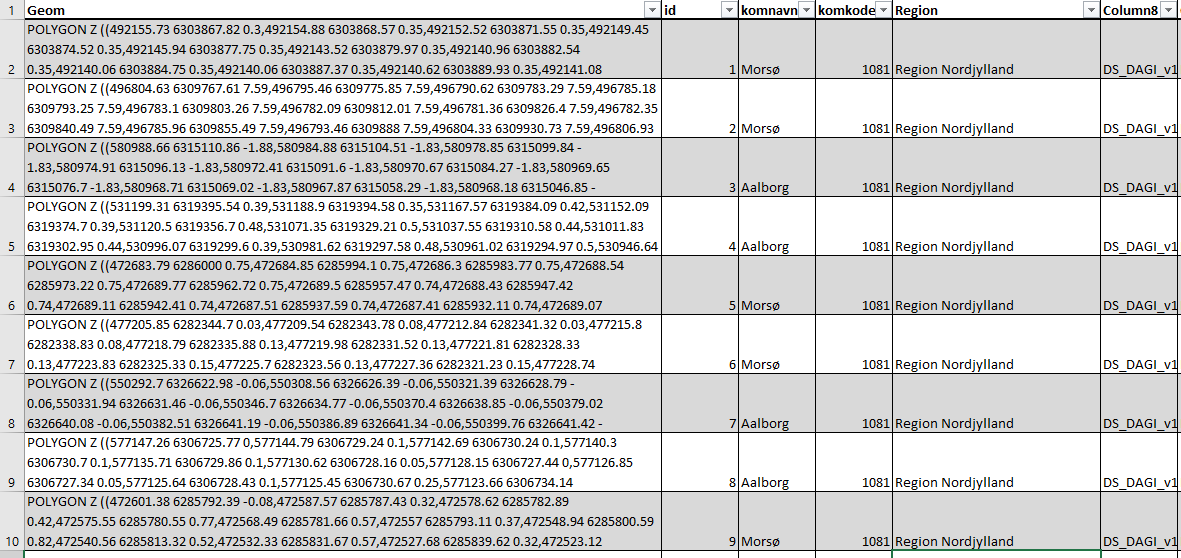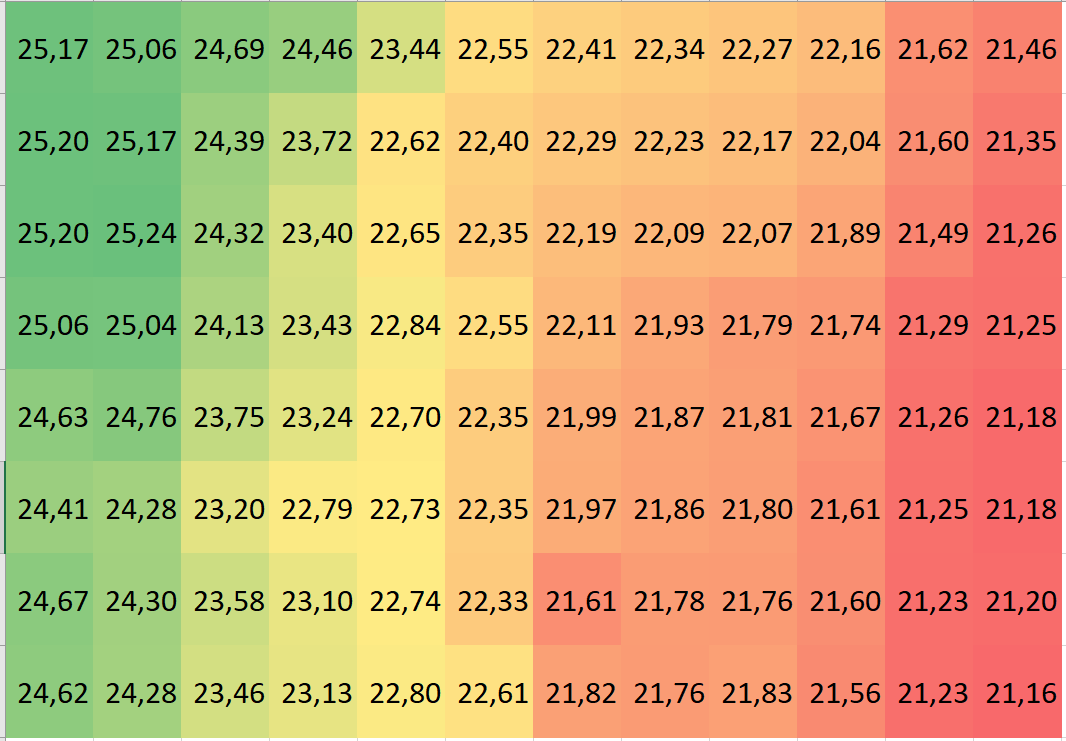# Introduction to Geospatial Data

Home » Learning paths » Introduction to Working with Geospatial Data » Introduction to Geospatial Data

The following is a simplified presentation of what GeoSpatial Data is and how it represents phenomena of interest. The purpose of this introduction is to give you just enough theoretical knowledge in order to follow the more practical learning path “How to make a map” or the more comprehensive presentation of the subject in the section “How to Represent Phenomena using Geospatial Data”

Stated simply, spatial data is data where the locationnal aspect (the where) plays a key role in the use of the data. GeoSpatial data is spatial data where the location is on the earth (Geo from Greek for earth or land). i.e. not on Mars or in J.R.R. Tolkien’s middle earth.

It is common to talk about three aspects of geospatial data namely:

• Attribute data
Represents the what or the properties of the geospatial information.
• Locational or positional data
Describes the “where” aspect of the geospatial information.
• Temporal data
Temporal data, if used, define a period in time that the combination of attribute and locational data relates to.

There are two fundamentally different use cases when it comes to geospatial data, namely representation of entities or representation of fields. An entity is in this context defined as something, for instance, a building that has a well-defined extent in time and space and where the same attribute data can apply to the entire extent. A field is in this context defined as a property (attribute) that varies continuously as a function of the location, for instance, elevation above sea level.

In the case of entity-based geospatial data describing buildings, the attribute data could describe the material of the roof of the building. The locational data describes the location/extent of the building and finally, the temporal data describes the time period where there existed a building at a given location with the given roof material. The fact that the data describes buildings is often given implicitly through the name of the data set.

In the case of field-based geospatial data describing air temperature, the attribute data would be the temperature, the locational data would be the location whose temperature was as described by the attribute data and finally the temporal data should be the data and time of day the attribute data refer to. Here the temporal data is often given through the name of the data set I,e, mean air temperature August 2022.

## Storing geospatial data

In the following, I will discuss the two main principles used to store geospatial data on a computer, namely vector and raster storage. It is worth noting that both entity and field bases geospatial data can be stored using either the raster or vector storage approach. However, there is a tendency for field-based representation to use the raster storage approach and entity-based representations to use the vector storage approach, but the choice of storage approach depends on many different factors

### Vector storage.

The principle of vector storage or as it is commonly named vector data is to represent the locational aspect using one of three geometry types: points, lines and polygons.

#### Points

In an entity-based representation, points are used to represent entities where the extent is considered to be of less importance, typically because it, at the intended scale of use is too small to be of relevance. This could for instance be lampposts, trees or boreholes.

In a field-based representation, points are used to define locations of known attribute values, i.e locations where the elevation above sea level has been measured.

As geometry points are given as (x,y) or (x,y,z) i.e. in either 2 or 3 dimensions where the z typically is the elevations above sea level.

``````p1 = Point(0,0)
p2 = Point(0,10)
p3 = Point(10,10)
p4 = Point(10,0)
p5 = Point(5,5)``````A simple plot of 5 points. You can experiment with the plots in this section by clicking the image (Will open a Python Notebook in Google Colab)

A common example of (x,y,z) data is elevation data collected using Lidar where a laser beam from an aeroplane or drone is bounced off the surface of the earth. The output of this method is a collection of (x,y,z) points where z is the elevation above sea level and the attributes among other things include the strength of the returned laser beam. The strength of the returned laser beam can be used to determine the type of surface, brick, soil, vegetation, etc. that reflected the laser beam. This process of using Lidar to determine the election is discussed more in the section on Lidar

#### Lines

In an entity-based representation, lines are used to represent linear features where the extent in terms of width is of less importance at the internet scale of use. Lines are typically used to for streams, power lines, railroads, roads etc. Roads are a bit of a special case in relation to both route planning and related elements such as bicycle paths and pavements. This is covered in the section on working with transport networks.

In a field-based representation, lines are used to represent lines of an equal attribute value, so-called isolines for instance Contour lines in an elevation data set or Isobars in an air pressure data set. The use of lines in field-based representations are not common and are primarily used for presentation purposes, i.e. human-readable maps rather than computer-based storage.

As geometry lines are represented as a series of points implicitly connected by straight lines segments i.e a line represented by the points (0,0) and (10,10) also includes the point (5,5)

``line = LineString([p1,p3])``A simple line from (0,0) to (10,10) includes the point (5,5). You can experiment with the plots in this section by clicking the image (Will open a Python Notebook in Google Colab)

#### Polygons

In an entity-based representation, polygons are used to represent entities, where not only the location but also the extent of the entity is important. This could for instance be buildings, forests, municipality etc.

In a field-based representation polygons are used to represent areas with a known constant attribute value for instance lakes in an elevation data set. As with lines, polygons are seldom used in field-based representation

As geometry polygons are represented as series of points implicitly connected by state line segments.

``poly = Polygon([p1,p2,p3,p4])``

Strictly speaking it is not quite correct to talk about polygons, since in geodata “polygons” can contain both holes and disconnected areas as can be seen below, however, I will stick to the naming practice and call them polygons.In geospatial data, polygons are used as a term that covers disconnected areas that even can include holes.

#### Datasets and attributes

Until now, we have looked at the individual geometries that are used in vector storage, In praxis, these geometries are organized into tables (like spreadsheets) where each attribute is represented by a column and the locational data is represented in an additional column, see Figure 1Figure 1 Vector data represented in an attribute table, The coordinates are typically not on display but in some applications such as PostGIS you can ask for the coordinates to be displayed as text.

### Raster storage

Raster data can be envisioned as a grid of cells where each cell can contain a single numerical value typically representing a single attribute e.g. elevation in meters over sea level.Figure 2 Elevation (property field)is represented as isolines (vector data). button elevation (property field)represented as Raster data (Each cell is 10 * 10 Meters) the numbers are elevation in meters over sea level.

The locational data is given through a combination of the position of the grid cell within the grid, and two additional pieces of information namely the real-world distance between the centre of each cell (in the case of figure 2 10 meters) and a real-world coordinate of a well-defined cell in the grid cell (typically the first cell in the first row)

In entity-based representations, entities are either represented by one or more cells containing an identifier of the entity or more commonly by a code number representing the type of the entety covering the individual cell e.g. 1 = forest, 2 = building 3 = lake.

In field-based representations, each cell contains the value of the attribute being represented at a well-defined location within the cell, typically the centre.

## Coordinate reference System

Common for both vector and raster data is that it needs a Coordinate Reference System (CRS) that maps the coordinates used in the geospatial data to a location on earth. The CRS is typically defined by giving an ESPG code which is a code value that refers to a well-defined list of CRS definitions. You can read more about how Coordinate Reference Systems work in more detail in the section on “Coordinate Reference Systems

## What next

After completing this section I would recommend one of two options to continue. You can either go on to the next section (How phenomena are represented as geospatial data) in the How geospatial data work learning path that covers more or less the same but in much more detail or you can get your feet wet and try making a map with the How to make a map learning path.